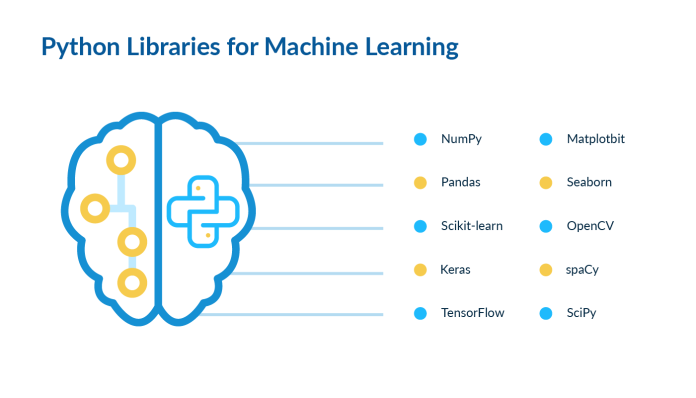# What are the popular Python libraries for Machine Learning?

0
335Machine Learning Machine Learning is a part of Artificial learning and is the core of computer processing, where computers learn without constantly giving commands by analyzing past data and predicting results. Traditionally, humans sorted and analyzed data to generate value from such data, which was a time-consuming and tedious process. There were always chances of error because of human limitations. But with the introduction of artificial intelligence and machine learning, the same process can be done in less time with Python libraries and with greater efficiency by programming the machine.

What is a Python library, and how does it work?

Python Library is a compilation of code used for different programs. It makes the work easy and convenient by eliminating the need for doing the same coding again and again. It works as a reusable bundle of codes and contains some built-in models that provide direct access to various functions. Such libraries are most commonly used for data science, artificial intelligence, and machine learningIn simple language, the Python library is an aid to machine learning which makes the learning process easy for machines.

Some commonly used Python libraries for machine learning are:

• TensorFlow: This library could be used for high-level computations and mathematics and Physics problems.
• PyTorch: It is easy to learn and use hence beginner-friendly. PyTorch is lighter to work with in comparison to TensorFlow,
• NumPy: It is used commonly for mathematical operations.
• Scipy: SciPy is a free and open-source Python library that provides structures for problems like integration-differentiation, interpolation, extrapolation, etc.
• Scikit-learn: It is the most famous Python library to work with data in the form of clusters.
• Keras and Theano: It is helpful in deep learning projects, and the speed with which it executes projects is Faster than TensorFlow.
• Pandas: It is an essential library that provides flexibility in operations and a wide variety of analytical tools.
• Matplotlib: Matplotlib is a library for creating animated and compelling visualizations in Python, making things easy to understand and more attractive.
• OpenCV: OpenCV is a library of programming operations mainly focused on processing data in real-time.
• Catboost: Catboost is an algorithm for decision making, flow charts, decision trees. This framework provides structure to solve out-of-the-box operations.
• fastText: fastText is a library for learning created by Facebook’s AI Research lab, supporting supervised and unsupervised learning.
• Chainer: Chainer is an open-source deep learning framework developed by a Japanese venture company.
• Matplotlib:  is used to plot numerical data in forms or pie charts and bar graphs to make the data understandable and presentable.